# NCERT Exemplar Class 10 Science Chapter 1 Chemical Reactions And Equations

NCERT Exemplar Class 10 Science Chapter 1 Chemical Reactions And Equations are part of NCERT Exemplar Class 10 Science. Here we have given NCERT Exemplar Class 10 Science Chapter 1 Chemical Reactions And Equations.

## NCERT Exemplar Class 10 Science Chapter 1 Chemical Reactions And Equation

1.Write the balanced chemical equations for the following reactions and identify the type of reaction in each case.
(a) Thermit reaction, iron (III) oxide reacts with aluminium and gives molten iron and aluminium oxide.
(b) Magnesium ribbon is burnt in an atmosphere of nitrogen gas to form solid magnesium nitride.
(c) Chlorine gas is passed in an aqueous potassium iodide solution to form potassium chloride solution and solid iodine.
(d) Ethanol is burnt in air to form carbon dioxide, water and releases heat.
Ans.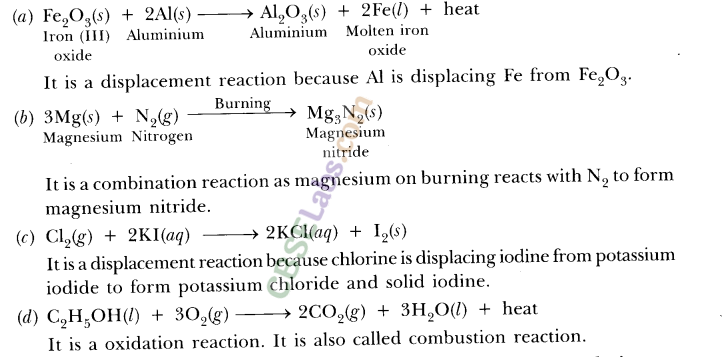2.A solution of potassium chloride when mixed with silver nitrate solution, an insoluble white substance is formed. Write the chemical reaction involved and also mention the type of the chemical reaction.
Ans.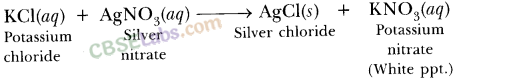It is a double displacement reaction. It is also a precipitation reaction as AgCl is a white precipitate.

3.Write the balanced chemical equations for the following reactions.
(a)Sodium carbonate on reaction with hydrochloric acid in equal molar concentrations gives sodium chloride and sodium hydrogen-carbonate.
(b)Sodium hydrogencarbonate on reaction with hydrochloric acid gives sodium chloride, water and liberates carbon dioxide.
(c)Copper sulphate on treatment with potassium iodide precipitates cuprous iodide $(Cu_{ 2 }{ I }_{ 2 })$, liberates iodine gas and also forms potassium sulphate.
Ans.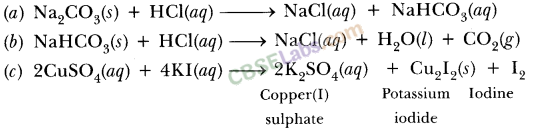4.Why do fireflies glow at night?
Ans. It is because protein present in fireflies undergoes oxidation in presence of air and an enzyme. This chemical reaction involves emission of visible light. Therefore, fireflies glow at night.

5.Grapes hanging on the plant do not ferment but after being plucked from the plant can be fermented. Under what conditions do these grapes ferment? Is it a chemical or a physical change?
Ans. Grapes when attached to plants are living and therefore they have their immune system due to which they cannot get fermented. When microbes attack plucked grapes in absence of air, they undergo fermentation to form alcohol. This is a chemical process.

6.During the reaction of some metals with dilute hydrochloric acid, following observations were made.
(a) Silver metal does not show any change.
(b) The temperature of the reaction mixture rises when aluminium (Al) is added.
(c) The reaction of sodium metal is found to be highly explosive.
(d) Some bubbles of a gas are seen when lead (Pb) is reacted with the acid. Explain these observations giving suitable reasons.
Ans. (a) It is because silver is less reactive than hydrogen. It cannot displace hydrogen from dilute acid.
(b) It is because the reaction is exothermic.
(c) It is because sodium is highly reactive and forms hydrogen gas in the presence of moisture $(H_{ 2 }O)$ which catches fire as the reaction is highly exothermic and $H_{ 2 }$ is highly inflammable.
(d) It is due to formation of hydrogen gas. The reaction becomes slow after some time as $PbCl_{ 2 }(s)$ covers Pb metal.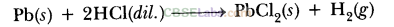7.A substance X, which is an oxide of a group 2 element, is used intensively in the cement industry. This element is present in bones also. On treatment with water, it forms a solution which turns red litmus blue. Identify X and also write the chemical reactions involved.
Ans.The substance ‘X’ is calcium oxide (CaO), element is calcium. Calcium oxide is used in cement industry. Calcium is present in bones in form of calcium phosphate.
Calcium oxide dissolves in water forming alkali which turns red litmus blue. CaO(s)+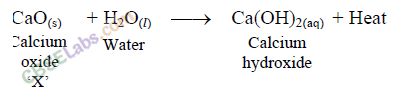8.Why do we store silver chloride in dark coloured bottles?
Ans.It is because silver chloride decomposes to silver and chlorine gas in presence of sunlight.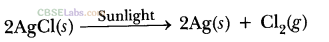9.A magnesium ribbon is burnt in oxygen to give a white compound X accompanied by emission of light. If the burning ribbon is now placed in an atmosphere of nitrogen, it continues to burn and forms a compound Y.
(а)Write the chemical formulae of X and Y.
(b)Write a balanced chemical equation, when X is dissolved in water.
Ans.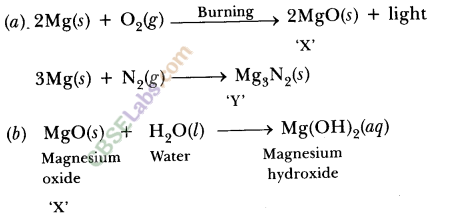10.A silver article generally turns black when kept in the open for a few days. The article when rubbed with toothpaste again starts shining.
(a) Why do silver articles turn black when kept in the open for a few days? Name the phenomenon involved.
(b) Name the black substance formed and give its chemical formula.
Ans.(a) Silver reacts with ${ H }_{ 2 }S$ gas present in atmosphere to form a black compound ${ Ag }_{ 2 }S$ (silver sulphide) on its surface. This phenomenon is called corrosion.
(b) ${ Ag }_{ 2 }S$(silver sulphide) is a black coloured solid.

11.Write the balanced chemical equation for the following equations for the following reaction and identify the type of reaction in each case.
(a)Nitrogen gas is treated with hydrogen gas in the presence of a catalyst at 773 K to form ammonia gas.
(b)Sodium hydroxide solution is treated with acetic acid to form sodium acetate and water.
(c)Ethanol is warmed with ethanoic acid to form ethyl acetate in presence of cone. ${ H }_{ 2 }SO_{ 4 }$.
(d)Ethene is burnt in presence of oxygen to form carbon dioxide, water and releases heat and light.
Ans.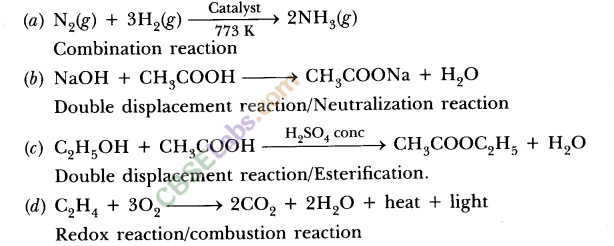12.Complete the missing components/variables given as x and y in the following reactions :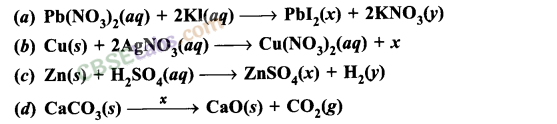Ans.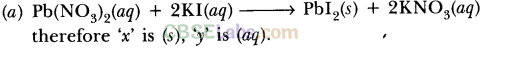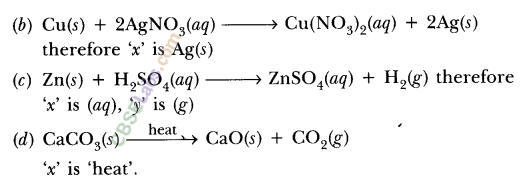13.Which among the following changes are exothermic or endothermic in nature?
Ans.(a)Decomposition of ferrous sulphate
(b)Dilution of sulphuric acid
(c)Dissolution of sodium hydroxide in water
(d)Dissolution of ammonium chloride in water Ans. (a) It is endothermic reaction.
(b)It is exothermic process.
(c)It is exothermic process.
(d)It is endothermic process.

14. Identify the reducing agent in the following reactions :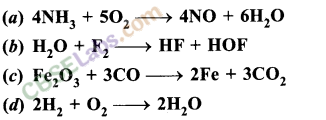Ans.(a)$HN_{ 3 }$ is reducing agent.
(b)$F_{ 2 }$ is reducing agent.
(c)CO (Carbon monoxide) is reducing agent.
(d)$H_{ 2 }$ is reducing agent.

15.Identify the oxidising agent (oxidant) in the following reactions :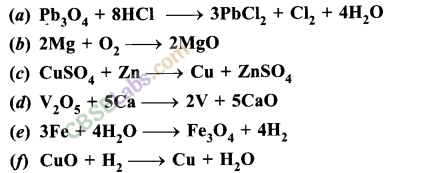Ans.(a)${ Pb }_{ 3 }O_{ 4 }$ (Red lead). It is also called Sindur used by married ladies. It is an oxidant (oxidising agent).
(b)$O_{ 2 }$ is oxidising agent.
(c)$CuSO_{ 4 }$ is oxidising agent.
(d)${ V }_{ 2 }O_{ 5 }$ is oxidising agent.
(e)$H_{ 2 }O$ is oxidising agent.
(f)CuO  is oxidising agent.

16.Ferrous sulphate decomposes with the evolution of gas having a characteristic order of burning sulphur. Write the chemical reaction involved and identify the type of reaction.
Ans.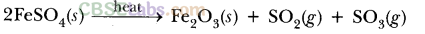It is decomposition reaction.

17.Which among the following are physical or chemical changes
(a)Evaporation of petrol.
(b)Burning of liquefied petroleum gas (LPG).
(c)Heating of an iron rod to red hot.
(d)Curdling of milk.
(e)Sublimation of solid ammonium chloride
Ans.(a)Physical change
(b)Chemical change
(c)Physical change
(d)Chemical change
(e)Physical change

18.Write a balanced chemical equation for each of the following reactions and also classify them.
(a)Lead acetate solution is treated with dilute hydrochloric acid to form lead chloride and acetic acid solution.
(b)A piece of sodium metal is added to absolute ethanol to form sodium ethoxide and hydrogen gas.
(c)Iron (III) oxide on heating with carbon monoxide reacts to form solid iron and liberates carbon dioxide gas.
(d)Hydrogen sulphide gas reacts with oxygen gas to form solid sulphur and liquid water.
Ans.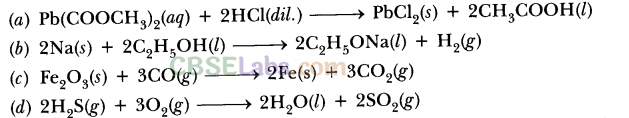19.Balance the following chemical equations arid identify the type of chemical reaction: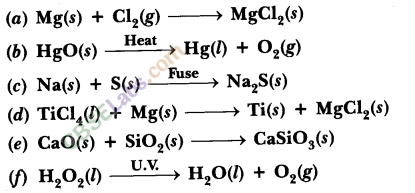Ans.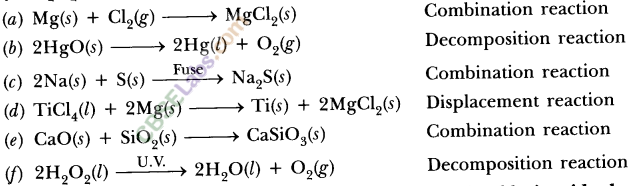20.Zinc liberates hydrogen gas when reacted with dilute hydrochloric acid, whereas copper does not. Explain why?
Ans.Zinc is more reactive than hydrogen whereas copper is less reactive than hydrogen. Therefore, Zn displaces hydrogen from dil.HCl whereas copper does not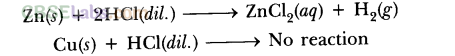21.On heating blue coloured powder of copper (II) nitrate in boiling tube, copper oxide (black), oxygen gas and a brown gas ‘X’ is formed.
(a)Write a balanced chemical equation of the reaction.
(b)Identify the brown gas ‘X’ evolved.
(c)Identify the type of reaction.
(d)What could be the pH range of aqueous solution of the gas ‘X’?
Ans.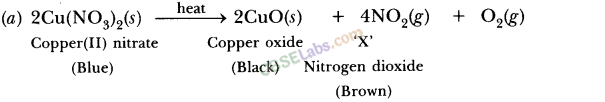(b) The brown gas ‘X,’ is nitrogen dioxide $(NO_{ 2 })$.
(c) The reaction is a thermal decomposition reaction.
(d) The gas ‘X’ is acidic in nature because it is a non-metallic oxide. Its aqueous solution has pH less than 7.

22.Give the characteristic tests for the following gases.
(a) $CO_{ 2 }$ (b) $SO_{ 2 }$ (c) $O_{ 2 }$(d) $H_{ 2 }$
Ans.(a)Pass the gas through limewater. Limewater turns milky which confirms the presence of $CO_{ 2 }$.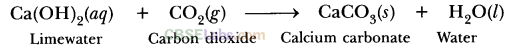Limewater Carbon dioxide Calcium carbonate Water
(b) Pass the gas through acidified potassium dichromate solution (orange in colour). The solution will become green in colour due to formation of$Cr_{ 2 }(SO_{ 4 })_{ 3 }$. It is a redox reaction. Acidified ${ K }_{ 2 }Cr_{ 2 }O_{ 7 }$ is oxidising agent whereas SO_{ 2 } is reducing agent.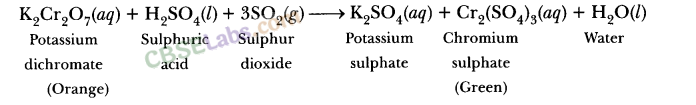Alternative:
Pass the gas through acidified potassium permanganate solution (pink/purple in r colour). It will become colourless because $SO_{ 2 }$ reduces $KMnO_{ 4 }$ to$Mn_{ 2 }+$ion which is colourless. It is a redox reaction. Acidified$KMnO_{ 4 }$is an oxidising agent.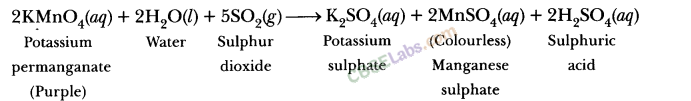(c) Bring a candle near oxygen gas. The intensity of candle flame is increased, it shows the presence of oxygen gas which is a supporter of combustion.
(d) Bring a burning matchstick near hydrogen gas. The gas will burn explosively ‘ with a pop sound. It confirms the presence of hydrogen.

23.What happens when a piece of
(a)zinc metal is added to copper sulphate solution?
(b)aluminium metal is added to dilute hydrochloric acid?
(c)silver metal is added to copper sulphate solution? Also, write the balanced chemical equation if the reaction occurs.
Ans.(a)The solution will become colourless due to formation of zinc sulphate and reddish brown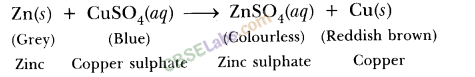(b)aluminium chloride solution will be formed.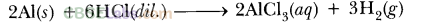(c) No reaction will take place because silver is less reactive than copper, it cannot displace copper from copper sulphate.

24.What happens when zinc granules are treated with dilute solutions of $H_{ 2 }SO_{ 4 }$, HCl, $HNO_{ 3 }$, NaCl and NaOH? Also write the chemical equations if reaction, occurs.
Ans.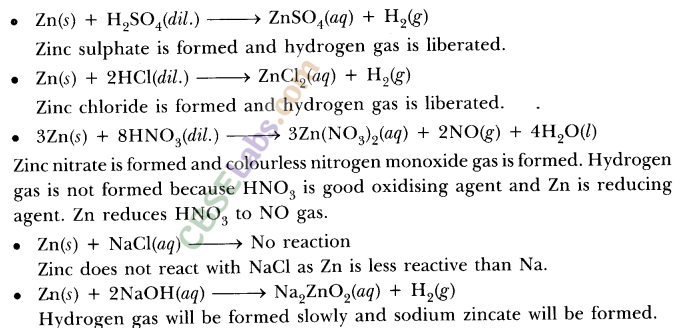25.On adding a drop of barium chloride solution to an aqueous solution of sodium sulphite, white precipitate is obtained.
(a)Write a balanced chemical equation of the reaction involved.
(b)What other name can be given to this precipitation reaction?
(c)On adding dilute hydrochloric acid to the reaction mixture, white precipitate disappears. Why?
Ans.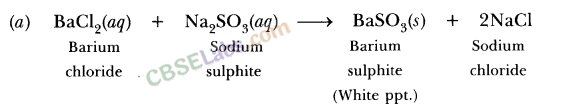(b)It is also called double displacement reaction.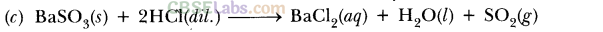It is because barium sulphite reacts with HC1 to form barium chloride which is soluble in water and liberates $SO_{ 2 }(g)$.

26.You are provided with two containers made up of (A) copper and (B) aluminium. You are also provided with solutions of (a) dilute HCl (b) dilute $HNO_{ 3 }$(c) ZnCl2 and (d) H20. In which of the above containers these solutions can be kept?
Ans.A. When solutions are kept in copper container-
(a)Dilute HC1: No reaction takes place as copper is less reactive than hydrogen. Therefore, dil. HC1 can be kept in copper container.
(b)Dilute HNOs: Copper will react with dil. HNOs as it is an oxidising agent and will form copper nitrate and nitrogen monoxide gas.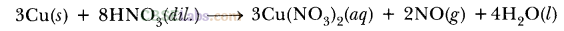Therefore, $HNO_{ 3 }$(dil.) cannot be kept in copper container.
(c) $ZnCl_{ 2 }$: No reaction takes place because copper is less reactive than Zn, therefore $ZnCl_{ 2 }$ can be kept in copper container.
(d)$H_{ 2 }O$ : NO reaction takes place because copper is less reactive than hydrogen. Therefore, water can be kept in copper container.
Thus, dil. HCl, $ZnCl_{ 2 }$ and $H_{ 2 }O$  can be kept in copper container whereas dil. $HNO_{ 3 }$ cannot be kept in it.
B.When solutions are kept in aluminium container-
(a)Dilute HCl: Aluminium reacts with dil. HCl to form aluminium chloride . and hydrogen gas is formed.
$2Al(s)+6Hcl(dil.)\longrightarrow 2AlCl_{ 3 }(aq.)+3H_{ 2 }(g)$
So, dil. HCl cannot be kept in aluminium container.
(b)Dilute $HNO_{ 3 }$: Aluminium gets oxidised by $HNO_{ 3 }$ to form a layer of aluminium oxide which does not react further therefore dil. $HNO_{ 3 }$can be kept in aluminium container.
(c) $ZnCl_{ 2 }$: It reacts with ‘AT to form aluminium chloride and zinc metal will get deposited.
$3ZnCl_{ 2 }+2Al\longrightarrow 2AlCl_{ 3 }+3Zn$
Therefore, Zinc chloride cannot be stored in aluminium container.
(d)$H_{ 2 }O{ 2 }$ : It can he stored in aluminium container because aluminium does not react with cold as well as hot water.
A1 reacts with steam to form aluminium oxide and hydrogen
$2Al(s)+3H_{ 2 }O(g)\longrightarrow Al_{ 2 }O_{ 3 }(s)+3H_{ 2 }( g)$
Thus.dil. $HNO_{ 3 }$ and $H_{ 2 }O$  can be kept in aluminium container whereas dil. HCl and $ZnCl_{ 2 }$ solution cannot be stored in it.

We hope the NCERT Exemplar Class 10 Science Chapter 1 Chemical Reactions And Equations help you. If you have any query regarding NCERT Exemplar Class 10 Science Chapter 1 Chemical Reactions And Equations, drop a comment below and we will get back to you at the earliest.